Open in App
Not now

# Class 8 RD Sharma Solutions- Chapter 18 Practical Geometry – Exercise 18.1

• Last Updated : 06 May, 2021

### Question 1: Construct a quadrilateral ABCD in which AB = 4.4 cm, BC = 4 cm, CD = 6.4 cm, DA = 3.8 cm and BD = 6.6 cm?

Solution:

Given: AB = 4.4 cm, BC = 4 cm, CD = 6.4 cm, DA = 3.8 cm and BD = 6.6 cm.

Let’s divide the quadrilateral into two triangles,

i.e., ΔABD and ΔBCD

Steps for the construction of a quadrilateral:

Step 1: Applying SSS congruency rule, Draw line BD of length 6.6 cm.

Step 2: Now, cut an arc with B as the centre and radius BC = 4 cm. Similarly by taking D as centre and radius CD = 6.4 cm.

Step 3: Join the intersection point from B and D and mark it as C.

Step 4: Now for vertex A, cut an arc by taking B as the centre and radius BA = 4.4 cm. Similarly by taking D as centre and radius DA = 3.8 cm.

Step 5: Join the intersection point from B and D and mark it as A.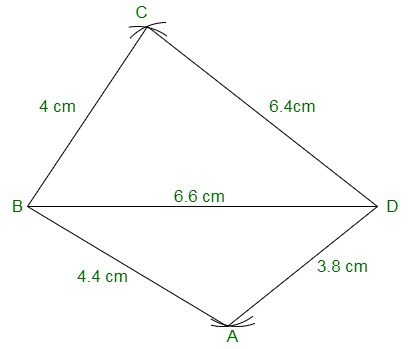### Question 2: Construct a quadrilateral ABCD in which AB = BC = 5.5 cm, CD = 4 cm, DA = 6.3 cm, AC = 9.4 cm Measure BD?

Solution:

Given: AB = BC = 5.5 cm, CD = 4 cm, DA = 6.3 cm, AC = 9.4 cm

We have to measure BD.

Steps for construction of the quadrilateral:

Step 1: Draw a line segment AB = 5.5 cm

Step 2: With B as centre and radius BC = 5.5 cm cut an arc. Mark the intersection point as C.

Step 3: With A as centre and radius AC = 9.4 cm cut an arc to intersect at point C.

Step 4: With C as centre and radius CD = 4 cm cut an arc. Mark the intersection point as D.

Step 5: With A as centre and radius AD = 6.3 cm cut an arc to intersect at point D.

Step 6: Now join BC, CD, and AD

Hence, the Measure of BD is 5.1 cm.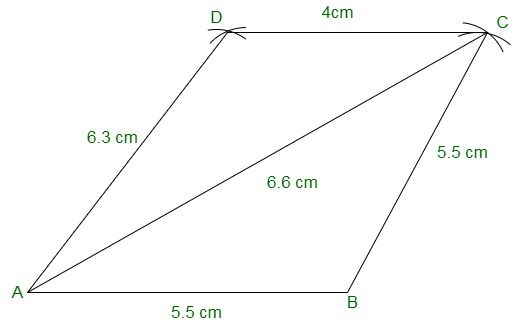### Question 3: Construct a quadrilateral XYZW in which XY = 5 cm, YZ = 6 cm, ZW = 7 cm, WX = 3 cm and XZ = 9 cm?

Solution:

We have, XY = 5cm, YZ = 6cm, ZW = 7cm, WX = 3cm and XZ = 9cm.

Step 1: Draw a line segment XZ with length = 9 cm.

Step 2: Taking X as the centre mark an arc of radius XY = 5 cm. Taking Z as centre and radius ZY = 6 cm, repeat the procedure.

Step 3: Mark the intersection point of X and Z as Y.

Step 4: For vertex W, taking X as the centre mark an arc of radius XW = 3 cm. Taking Z as centre and radius ZW = 7 cm, repeat the procedure.

Step 5: Mark the intersection point of X and Z as W.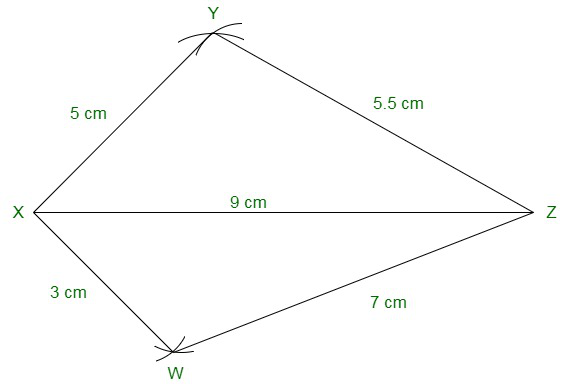### Question 4: Construct a parallelogram PQRS such that PQ = 5.2 cm, PR = 6.8 cm, and QS = 8.2 cm?

Solution:

We have, PQ = 5.2 cm, PR = 6.8 cm, and QS = 8.2 cm.

Steps to construct a parallelogram:

Step 1: Draw a line segment QS of length = 8.2 cm.

Step 2: Divide the line segment QS into two equal parts such that each one is = 4.1 cm. Mark the midpoint as O.

Step 3: Taking O as the centre, draw arcs of length 3.4 cm each on both the sides of the points O. Mark these points as P and R respectively

Step 4: Taking Q as a centre and radius QR = 5.2cm, draw an arc that intersects point R.

Step 5: Also, taking Q as a centre and radius QP = 5.2cm, again draw an arc that intersects point P.

Step 6: Join sides PQ, PS, QR, and RS to form a parallelogram.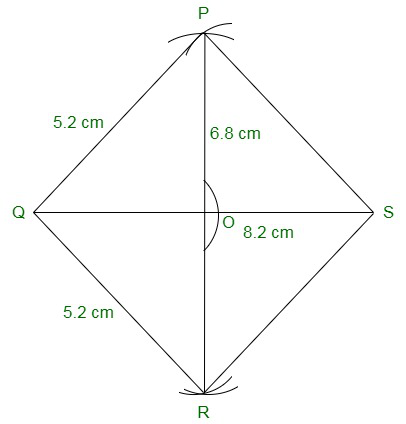### Question 5: Construct a rhombus with a side of 6 cm and one diagonal of 8 cm. Measure the other diagonal?

Solution:

In a rhombus, all the sides of a rhombus are equal and diagonals bisect each other.

We have a side 6 cm and one diagonal 8 cm.

Steps to construct a rhombus:

Step 1: Draw a line segment XZ of length 8 cm.

Step 2: Taking a radius = 6 cm, cut an arc with X as the centre. Repeat the procedure with Z as the centre and radius = 6 cm.

Step 3: Mark the intersection point of X and Z as Y.

Step 4: Taking a radius = 6 cm, cut an arc with W as the centre. Repeat the procedure with Z as the centre and radius = 6 cm.

Step 5: Mark the intersection point of X and Z as W.

Step 6: Join the line segments XY, XW, XZ, and ZY to form a rhombus.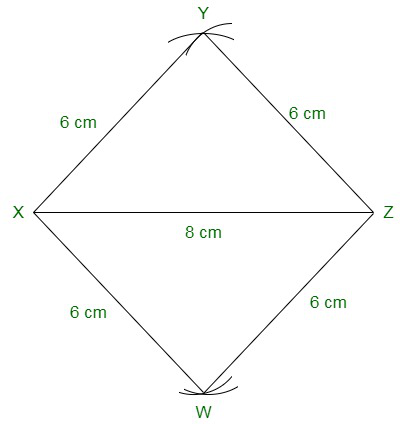### Question 6: Construct a kite ABCD in which AB = 4 cm, BC = 4.9 cm, AC = 7.2 cm?

Solution:

We have, AB = 4 cm, BC = 4.9 cm, AC = 7.2 cm.

Steps to construct a kite:

Step 1: Draw a line segment AC with length =  7.2 cm.

Step 2: Taking a radius of 4 cm and cut an arc by taking A as the centre. Also, taking C as the centre, repeat the procedure with radius = 4.9 cm.

Step 3: Label the intersection point of A and C and mark it as B.

Step 4: Considering vertex D, draw an arc with A as the centre. Also, taking C as the centre, repeat the procedure with radius = 4.9 cm.

Step 5: Label the intersection point of A and C and mark it as D.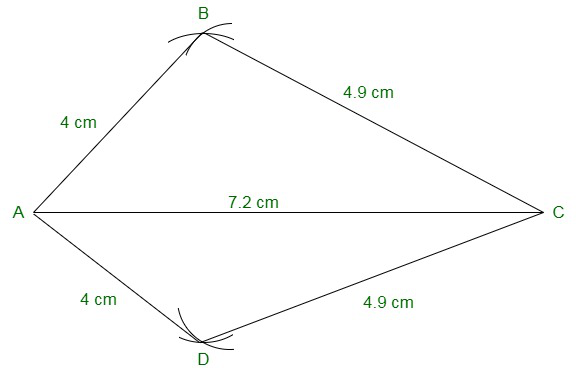### Question 7: Construct, if possible, a quadrilateral ABCD given AB = 6 cm, BC = 3.7 cm, CD = 5.7 cm, AD = 5.5 cm and BD = 6.1 cm. Give reasons for not being able to construct it, if you cannot?

Solution:

We have, AB = 6 cm, BC = 3.7 cm, CD = 5.7 cm, AD = 5.5 cm and BD = 6.1 cm.

Step 1: Draw a line segment AB with length = 6 cm.

Step 2: Taking A as the centre, draw an arc of a radius of 5.5 cm. Mark this point as D.

Step 3: Taking B as a centre, draw an arc of a radius of 6.1 cm intersecting point D.

Step 4: Also, with B as a centre, draw another arc of a radius of 3.7 cm. Mark this point as C.

Step 5: Taking D as a centre, draw an arc of a radius of 5.7 cm intersecting point C.

Step 6: Join the line segments, AD, BD, BC, and DC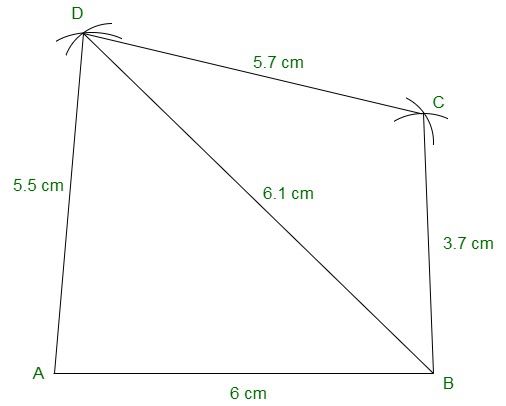### Question 8: Construct, if possible, a quadrilateral ABCD in which AB = 6 cm, BC = 7 cm, CD = 3 cm, AD = 5.5 cm and AC = 11 cm. Give reasons for not being able to construct, if you cannot. (Not possible, because in triangle ACD, AD + CD<AC)?

Solution:

We have, AB = 6 cm, BC = 7 cm, CD = 3 cm, AD = 5.5 cm and AC = 11 cm.

Since, we know, In any triangle the sum of the length of its two sides must be greater than that of the third side, therefore a quadrilateral with such dimensions cannot be constructed.

Considering in triangle ACD,

AD + CD = 5.5 + 3 = 8.5 cm

Given, AC = 11 cm

Now, AD + CD < AC which is not possible.

Hence, the construction cannot be performed.

My Personal Notes arrow_drop_up